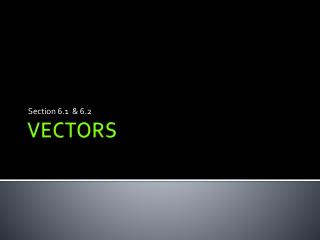# VECTORS - PowerPoint PPT PresentationDownload PresentationVECTORS

VECTORS
Download Presentation## VECTORS

- - - - - - - - - - - - - - - - - - - - - - - - - - - E N D - - - - - - - - - - - - - - - - - - - - - - - - - - -
##### Presentation Transcript

1. Section 6.1 & 6.2 VECTORS

2. “still” measurements “moving” measurements Force Velocity Acceleration • Temperature • Distance • Height • Area • Volume

3. Vector • A directed line segment (arrow) with its head tale at the origin and its head at the point (a, b) • Represents both direction & magnitude Vector with components a & b

4. Magnitude • The “amount of something” (i.e. force& speed) • Magnitude = length of the vector

5. Magnitude

6. Vectors that are the same length and the same direction are equivalent “component vector”

7. Ex 1) Draw the directed line segment from R(-4,7) to S(-1,5) Component Vector: Magnitude: Linear Combination:

8. Ex 2) Draw the directed line segment from P=(1,-2) to Q=(3,4) Component Vector: Magnitude: Linear Combination:

10. Scalar Multiplication

11. Dot Product • A way of “multiplying” vectors • Answer is a real number

12. Orthogonal: perpendicular (90°) • Dot product will equal ZERO u v

13. Ex 3) Perform each vector operation a.)u + v b.) 3u c.) u+(-2)v d.) u ∙ v

14. Ex 4) Are the vectors below orthagonal?

16. Ex 5) Add the Vectors Graphically

17. Ѳ is called the “direction angle” Magnitude y -vertical Ѳ x -horizontal

18. Ex 6) Find the horizontal &vertical components of vector vwith direction angle 25° and magnitude 18

19. Ex 7) Find the direction angle of the vector

20. “Magnitude” • Velocity (speed) • Gravity (force)

21. Ex 8)Hector is trying to get his dog to keep walking. He pulls on the leash with a force of 67 Newton's, at an angle of 30 degrees above the horizontal. Determine the vertical and horizontal components of the vector.

22. PRACTICE

23. What is the component form of the directed line segment from P(-1, 5) Q(4, 2)?

24. What is the magnitude of the vector

25. Write the vector below as a linear combination:

27. Determine if the vectors below are orthagonal

28. Find the sum of the vectors graphically

29. Find the horizontal & vertical components of a vector with a magnitude of 12 and direction angle of 35°

30. What is the direction angle of a vector with a magnitude of 10 and a horizontal component of 5?

31. 1. Helen is parasailing. She sits in a seat harness which is attached by a tow rope to a speedboat. The rope makes an angle of 51° with the horizontal and has a tension of 350 N. Determine the horizontal and vertical components of the tension force.

32. 2. A plane is flying on a bearing of 65° at 500 mph. Find the component form of the velocity of the airplane *Bearing is the angle formed with due north measure clockwise

33. Vector Equations of Lines Equation of a line passing through v

34. #12 a. (-2, 5) and (4, 2) b. (3, 4) and (6, -3)